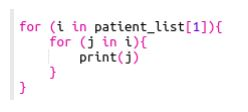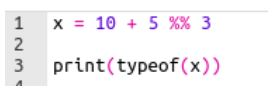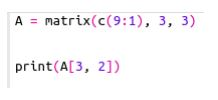# NPTEL Data Science for Engineers Assignment 1 Answers 2023

NPTEL Data Science for Engineers Assignment 1 Answers 2023:- All the Answers provided below to help the students as a reference, You must submit your assignment at your own knowledge.

## NPTEL Data Science For Engineers Week 1 Assignment Answers 2023

1. Which of the following variable names are INVALID in R?

• 1_variable
• variable_1
• _variable
• variable@
```Answer :- a. 1_variable
d. variable@

In R, variable names must follow certain rules and conventions. The valid variable names in R can only contain letters (a-z, A-Z), numbers (0-9), and dots (.) or underscores (_). Variable names cannot start with a number or contain special characters other than dots or underscores.```

2. The function ls() in R will

• set a new working directory path
• list all objects in our working environment
• display the path to our working directory
• None of the above
```Answer :- b. list all objects in our working environment

ls() stands for "list objects" and is used to display the names of objects (variables, functions, etc.) that are currently present in the working environment in R. It does not change the working directory or display the path to the working directory. Instead, it lists the objects available in the current R session.```

Consider a following code snippet. Based on this, answer questions 3 and 4.

3. Which of the following command is used to access the value “Shyam”?

• print(patient list)
• print(patient list[])
• print(patient list[])
• print(patient list[])
```Answer :- c. print(patient_list[])

In R, double square brackets [[ ]] are used to extract elements from a list. The first index [] selects the third element of the list, which is itself another list. Then, the second index  selects the second element from this inner list, which is "Shyam". Therefore, patient_list[] will give you the value "Shyam".```

4. The output of the code given below isd. Code will throw an error.

`Answer :- c. `

5. What is the output of following code?• double
• integer
• list
• None of the above
`Answer :- a. double`

6. State whether the given statement is True or False.
The library reshape2 is based around two key functions named melt and cast.

• True
• False
```Answer :- True.

The statement is true. The library reshape2 in R is built around two key functions: melt and dcast (formerly known as acast). These functions are used for reshaping data from wide to long format and vice versa. The melt function is used to transform data from a wide format to a long format, while dcast is used to transform data from a long format to a wide format.```

7. What is the output of following code?• 6
• 4
• 2
• 8
`Answer :- b. 4`

Create the data frame using the code given below and answer questions 8 and 9.

student data = data.frame(student id=c(1:4), student name=c(‘Ram’,‘Harish’,‘Pradeep’,‘Rajesh’))

8. Choose the correct command to add a column named student_dept to the dataframe student_data.

• student datastudent dept=c(“Commerce”, “Biology”, “English”, “Tamil”)
• student data[“student dept”]= c(“Commerce”,“Biology”, “English”,“Tamil”)
• student dept= student data[c(“Commerce”,“Biology”,“English”,“Tamil”)]
• None of the above
```Answer :- a. student_data\$student_dept = c("Commerce", "Biology", "English", "Tamil")

b. student data[“student dept”]= c(“Commerce”,“Biology”, “English”,“Tamil”)```

9. Choose the correct command to access the element Tamil in the dataframe student_data

• student data[]
• student data[]
• student data[]
• None of the above
```Answer :- student_data[]

In R, double square brackets [[ ]] are used to extract elements from a list or dataframe. In this case, student_data[] extracts the third column (which is a vector containing "Commerce", "Biology", "English", "Tamil"), and then  is used to access the fourth element in that vector, which is "Tamil". Therefore, student_data[] will give you the value "Tamil".```

10. The command to check if a value is of numeric data type is _____.

• typeof()
• is.numeric()
• as.numeric()
• None of the above
```Answer :- is.numeric()

The is.numeric() function in R is used to determine whether a given value is of numeric data type or not. It returns TRUE if the value is numeric and FALSE otherwise. For example:```

## NPTEL Data Science for Engineers Assignment 1 Answers 2022 [July 2022]

1. Choose the variable name(s) that are valid in R.

a. emp_name = “Ram”
b. emp.name = “Ram”
c. empname1 “Ram”
d. 1empname = “Ram”

`Answer:- a, b, c`

2. The function ls() in R will

a. set a new working directory path
b. list all objects in our working environment
c. display the path to our working directory
d. None of the above

`Answer:- b`

3. Consider the following code in R.

``If we supply 4 as an argument to the function find.function, after executing the above code, the result displayed would be ``
• the numbers “1,2,3,4”
• the numbers “1,4,9,16”
• the number “4”
• None of the above
`Answer:-b`

4. The next statement in R programming is useful

a. for skipping the current iteration of a loop without terminating it
b. for terminating the current iteration of a loop
c. for evaluating the current iteration of a loop without terminating it
d. None of the above

`Answer:- a`

Create the data frame using the code given below and answer questions 5 and 6

5. Choose the correct command to add a column named student_dept to the dataframe student_data

a. student_data\$student_dept=c(“Commerce”,”Biology”,”English”,”Tamil”)
b. student_data[“student_dept”]= c(“Commerce”,”Biology”,”English”,”Tamil”)
c. student_dept= student_data[c(“Commerce”,”Biology”,”English”,”Tamil”)]
d. None of the above

`Answer:- b`

6. Choose the correct command to access the element Tamil in the dataframe student_data

a. student_data[]
b. student_data[]
c. student_data[]
d. None of the above

`Answer:- c`

7. State whether the given statement is True or False.

The library reshape2 is based around two key functions named melt and cast.

a. True
b. False

`Answer:- a`

8. Consider the following code.

``If a room temperature of 20℃ is input to the above code, choose the output displayed ``
• “The room is cold. Please turn the heater on!”
• “The room is warm” “Enjoy the stay!”
• “The room is hot” “Please turn the air conditioning on!”
• None of the above
`Answer:- b`

9. Table 1 provides the scores of the students in three subjects. Create a data frame called students_scores out of Table 1. Which of the following options gives Table 2 as an output?

`Answer:- a`

10. The command to check if a value is of numeric data type is ___

`Answer:- b`

## What is Data Science for Engineers?

Data Science for Engineers is a fun-filled course where Domain Certification helps learners to gain expertise in a specific Area/Domain. This can be helpful for learners who wish to work in a particular area as part of their job or research or for those appearing for some competitive exam or becoming job-ready or specialising in an area of study.  Every domain will comprise Core courses and Elective courses. Once a learner completes the requisite courses per the mentioned criteria, you will receive a Domain Certificate showcasing your scores and the domain of expertise.

CRITERIA TO GET A CERTIFICATE

Average assignment score = 25% of the average of best 6 assignments out of the total 8 assignments given in the course.
Exam score = 75% of the proctored certification exam score out of 100

Final score = Average assignment score + Exam score

YOU WILL BE ELIGIBLE FOR A CERTIFICATE ONLY IF THE AVERAGE ASSIGNMENT SCORE >=10/25 AND EXAM SCORE >= 30/75. If one of the 2 criteria is not met, you will not get the certificate even if the Final score >= 40/100.

## NPTEL Data Science for Engineers Assignment 1 Answers 2022 [Jan 2022]

Q1. The function “ls ()” in R will

(A) set a new working directory path
(B) list all objects in our working environment
(C) display the path to our working directory
(D) list all files in our working directory

Ans:- (D) list all files in our working directory

Q2. Consider the following code:

If a room temperature of 20 degree Celsius is input to the above code, choose the output displayed:

(A) “The room is chilly. Please turn the heater on!”
(B) “The room is warm” “Enjoy the stay!”
(C) “The room is hot” “Please turn the air conditioning on!”
(D) None of the above

Ans:- (B) “The room is warm” “Enjoy the stay!”

Q3. Consider the code below to create a dataframe city_data:

Choose the correct answer based on the output expected on running the below code.

(A) In the column weather in dataframe city_data, “Rain” is replaced by “Snowy”
(B) In the column name in dataframe city_data, “City C” is replaced by “Snowy”
(C) No change occurs to the entries in the dataframe city_data
(D) The code raises an error

Ans:- (A) In the column weather in dataframe city_data, “Rain” is replaced by “Snowy”

Q4. Consider the code given below.

Choose the correct command to access the variable “Cloudy”

(A) city_weather[]
(B) city_weather[]
(C) city_weather[]
(D) None of the above

Ans:- (A) city_weather[]

Q5. Consider the following code.

A = matrix(c(1:42), nrow = 6, ncol = 7, byrow = T)
B = A[-2,]

Based on the output of the above code, choose the correct options from the following

(A) B is a matrix consisting of only elements of the second row of matrix A
(B) B is a matrix consisting of same elements as that of A
(C) The code raises an error
(D) B is a matrix consisting of all elements of A excluding the second row

Ans:- (D) B is a matrix consisting of all elements of A excluding the second row

Q6. The parameter “collapse” in the function paste()

(A) adjusts the display of the string to left, right or center
(B) add space in between two strings
(C) eliminates the space in between two strings
(D) eliminates the space within two words in a string

Ans:- (B) add space in between two strings

Q7. Consider the following code in R.

If we supply 4 as an argument to the function  find.function, after executing the above code, the result displayed would be

(A) the numbers “1,2,3,4”
(B) the numbers “1,4,9,16”
(C) the number “4”
(D) None of the above

Ans:- (B) the numbers “1,4,9,16”

Q8. The operator %in% is used for

(A) multiplication of a matrix with its transpose
(B) generating a sequence of numbers in a vector
(C) identifying if an element belongs to a vector
(D) none of the above

Ans:- (C) identifying if an element belongs to a vector

Q9. The output of compiling and executing the following code in R would be

(A) The term “Green” “apple” printed 5 times.
(B) The term “Green apple” printed 3 times.
(C) The term “Green” printed 4 times.
(D) The term “Green” “apple” printed 4 times.

Ans:- (D) The term “Green” “apple” printed 4 times.

Q10. The “next” statement in R programming is useful

(A) for skipping the current iteration of a loop without terminating it.
(B) for terminating the current iteration of a loop.
(C) for evaluating the current iteration of a loop without terminating it.
(D) none of the above

Ans:- (A) for skipping the current iteration of a loop without terminating it.

Disclaimer:- We do not claim 100% surety of solutions, these solutions are based on our sole expertise, and by using posting these answers we are simply looking to help students as a reference, so we urge do your assignment on your own.